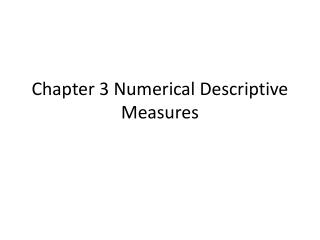DownloadDownload PresentationChapter 3 Numerical Descriptive Measures

# Chapter 3 Numerical Descriptive Measures

Download Presentation## Chapter 3 Numerical Descriptive Measures

- - - - - - - - - - - - - - - - - - - - - - - - - - - E N D - - - - - - - - - - - - - - - - - - - - - - - - - - -
##### Presentation Transcript

1. Chapter 3 Numerical Descriptive Measures

2. Contents • Central Tendency: the extent to which all of the data values group around a central value. • Variation: the amount of dispersion or scattering of values away from a central point • Shape: the pattern of the distribution of values from the lowest value to the highest value

3. Blackstone (BX) • Nov 28 2007 to Jan 17 2008

4. Central Tendency • Mean (arithmetic mean) • Is the sum of the values divided by the number of values. • Drawback of Mean (extreme values) • Median • The value that splits a ranked set of data into two equal parts. • Median = (n+1)/2

5. The mode • The value in a set of data that appears most frequently. • Quartiles • Split a set of data into four equal parts • Q1 = (n+1)/4 • i.e. n = 9 or n = 10

6. The Geometric Mean • Measures the rate of change of a variable over time • Is the nth root of the product of n values • i.e. Rate of return (Jan 7-Jan 8) = 7.2% Rate of return (Jan 8-Jan 9) = 7.9% What’s the rate of return from Jan 7-Jan 9?

7. Range • Range • Difference between the largest and the lowest • Interquartile Range • Difference between the third and first quartiles in a set of data (middle fifty)

8. Variance and standard deviation • Sample variance is the sum of the squared differences around the mean divided by the sample size minus one • Standard deviation is the square root of the variance • Why use standard deviation?

9. Coefficient of variation • Why is it important? • Z scores • Detect outliers

10. Shape • Symmetrical • Skewed • Left Skewed • Right Skewed • Symmetrical

11. 3.2 Numerical Description Measures For A Population • Population Mean • Population Standard Deviation and Variance

12. Empirical Rule • If distribution is symmetrical, population mean and stdv. can tell us a lot more about the distribution of the data • Example: Assume Blackstone stock follows a symmetrical distribution, what percent of stock price fall into the range between mean and first stdv.

13. The Chebyshev Rule • If the distribution is skewed, the percentage of values that are found within distance of k δ From the µ must be at least (1 – 1/k²) × 100%

14. 3.3 Computing Numerical Descriptive Measures From A Frequency Distribution • Reading Assignment PP99

15. 3.4 Exploratory Data Analysis • The Five Number Summary • Min, Quartile 1, Median, Quartile 2 and Max • Help us determine the shape of the distribution • i.e. The following data represent the total fat for burgers from a sample of fast-food chains. 19, 31, 34, 35, 39, 39 and 43

16. The Box-and-Whisker Plot • A graphical representation of the data based on the five number summary.

17. 3.5 The Covariance and The Coefficient of Correlation • The Covariance • Measures the strength of the linear relationship between two numerical variables. • Sample covariance

18. The coefficient of correlation • Measures the relative strength of a linear relationship between two numerical variables. • It ranges from -1 for a perfect negative correlation to +1 for a perfect positive correlation. Zero means no correlation.

19. The coefficient of correlation (Cont’d) • Sample coefficient of correlation